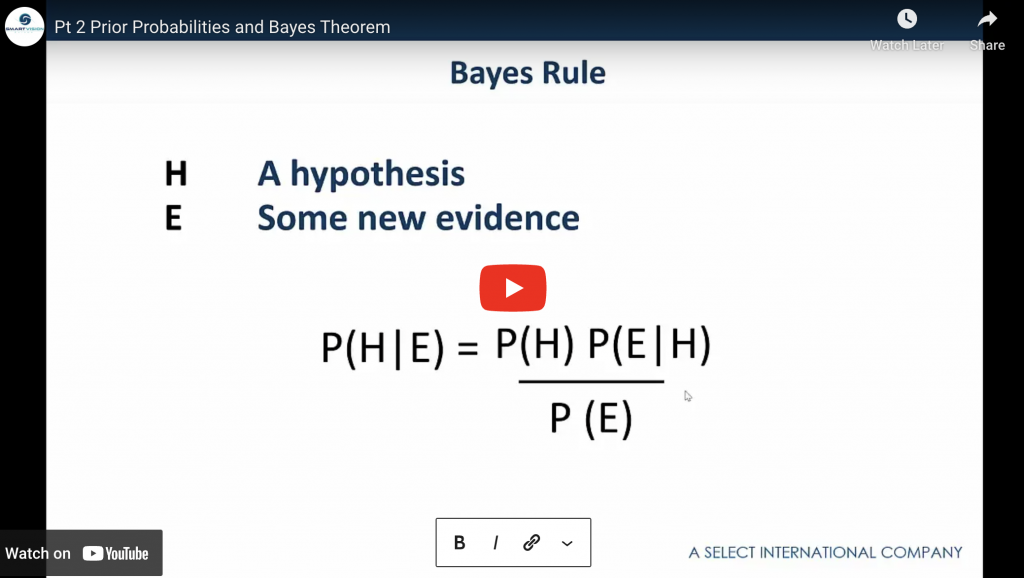# Performing Bayesian analysis in SPSS StatisticsIn this on demand webinar Jarlath Quinn explores Bayesian approaches to statistical analysis and shows how to perform and interpret a Bayesian tests in SPSS.

Content includes:

• The historical and theoretical context of the classical ‘Frequentist’ statistical approach and its Bayesian counterpart
• The concept of Prior Probabilities and how these are utilised in Bayes Theorem
• Credible intervals and the use of Bayes Factor as an alternative to P values
• How to perform a Bayesian Analysis in SPSS Statistics and how to interpret the output